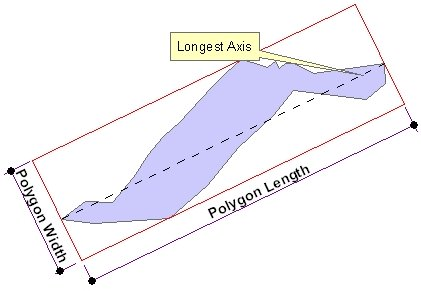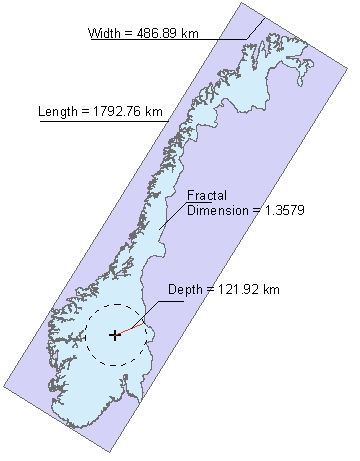Open Table of Contents

Polygon Characteristics

Go to ToolBox Implementation

Calculates some characteristics of the polygons from a polygon dataset

Inputs:

• A Polygon feature class

Outputs:

• The results can be added to the input feature class or a new polygon feature class. All attributes of the original features are preserved
• New fields added to the attribute table
• ET_Length - the length of the longest axis in the units of the Spatial Reference of the input feature class.
• ET Width - the length of shortest side of the bounding rectangle aligned with the longest axis in the units of the Spatial Reference of the input feature class.
• ET Circ - Circularity ratio - for a circle the circularity will be 1. The thinner the polygon is the smaller the circularity will be.
• ET Thick - Thickness ratio expressed as a ratio of the polygon area versus the area of its minimum bounding square. The ratio will have value of 1 for a square. The smaller the value is, the thinner the polygon is.
• ET_Parts - the number of parts that the polygon has
• ET_Holes - the number of holes in the polygon
• ET_HasArcs - if the polygon has true arc segments - 1 otherwise - 0
• ET_Vert - the number of vertices of the polygon
• ET_Depth - the distance from the deepest point (the center of the maximum inscribed circle) to the polygon boundary. See Polygon To Point and Polygon To Maximum Inscribed Circle functions
• ET_Fract - the fractal dimension (indication of the complexity) of the polygon boundary.  The value is between 1 and 2. The more complex the polygon boundary is the larger  the fractal dimension will be.

Notes:

• Fractal Dimension of the polygon boundaries is calculated using the Box Counting method (1)

• Calculating the Fractal Dimension and Polygon Depth is time consuming. If you don't need these characteristics, uncheck the options for faster processing.

Illustrations:References:

1. Bourke, P., 1993. Fractal Dimension Calculator User Manual, Online. Available: http://paulbourke.net/fractals/fracdim/

Command line syntax

ET_GPPolygonCharacteristics <input_dataset> {polygon_depth} {fractal_dimension} {precision}

Parameters

Expression Explanation
<input_dataset> A Point feature class or feature layer.
{fractal_dimension} A Boolean indicating whether to calculate polygon depth or not.
{polygon_depth} A Boolean indicating whether to calculate fractal dimension or not.
{precision} An Integer between 0 and 8 representing the number of places after the decimal point to be used.

Scripting syntax

ET_GPPolygonCharacteristics (input_dataset, polygon_depth, fractal_dimension, precision)

See the explanations above:
<> - required parameter
{} - optional parameter

 Copyright © Ianko Tchoukanski